# RD Sharma Solutions For Class 7 Maths Chapter 15 Properties of Triangles Exercise 15.2

Get free PDF of RD Sharma Solutions for Class 7 Maths Exercise 15.2 of Chapter 15 Properties of Triangles from the provided links. These PDF’s can be easily downloaded by the students. Our experts have uniquely formulated these questions to help the students gain competence. RD Sharma Solutions for Class 7 is one of the best study material designed for CBSE students. This exercise deals with the angle sum property of a triangle and some more results on triangular properties.

## Download the PDF of RD Sharma Solutions For Class 7 Maths Chapter 15 – Properties of Triangles Exercise 15.2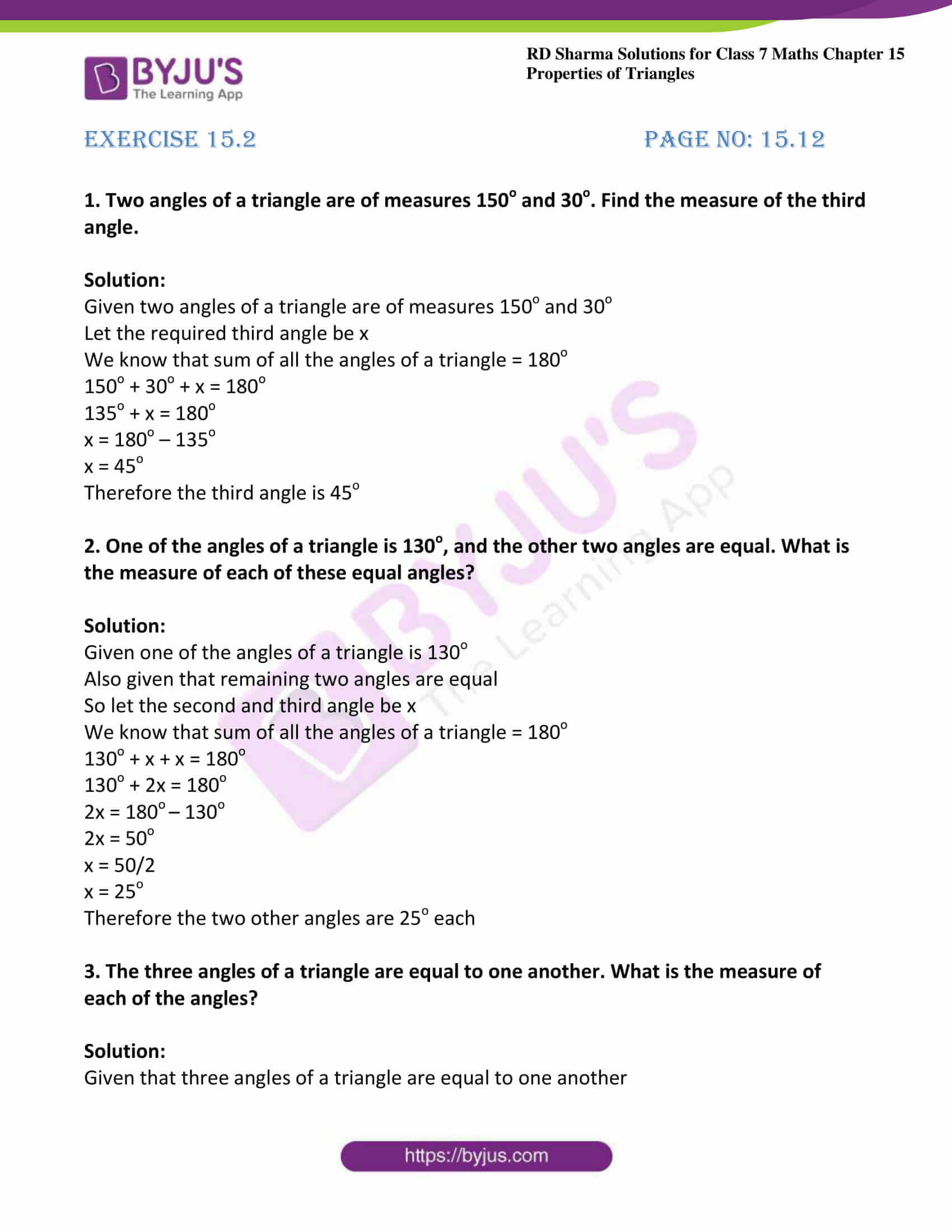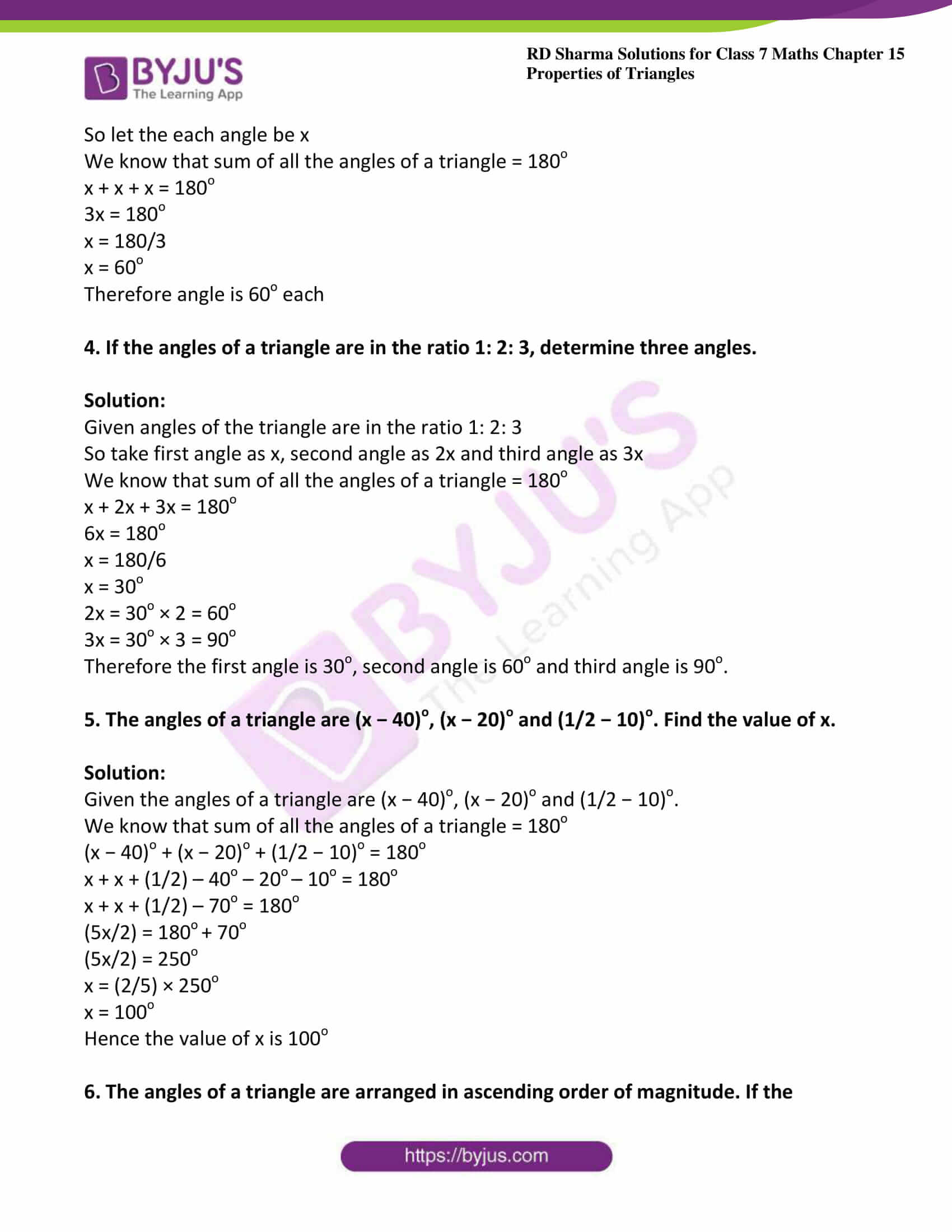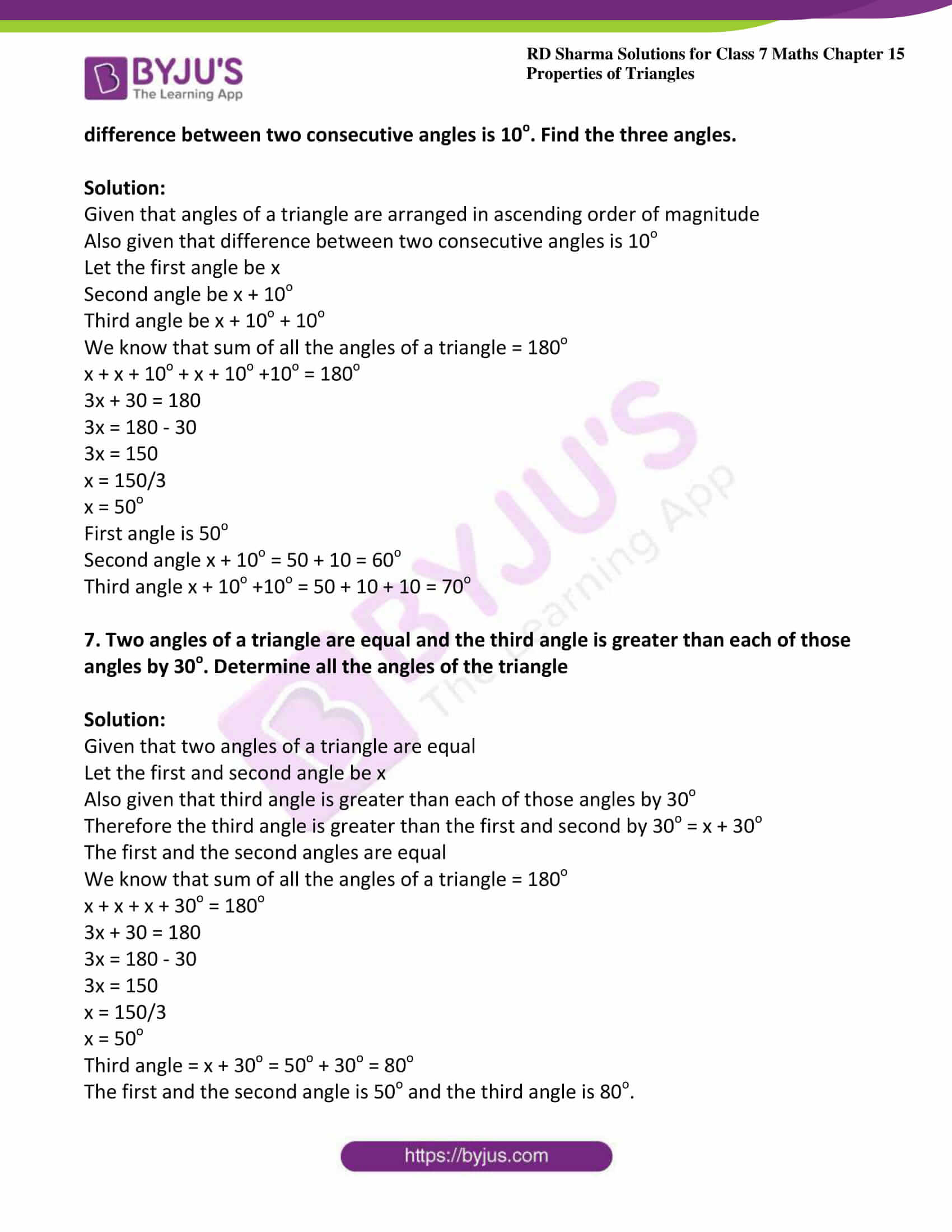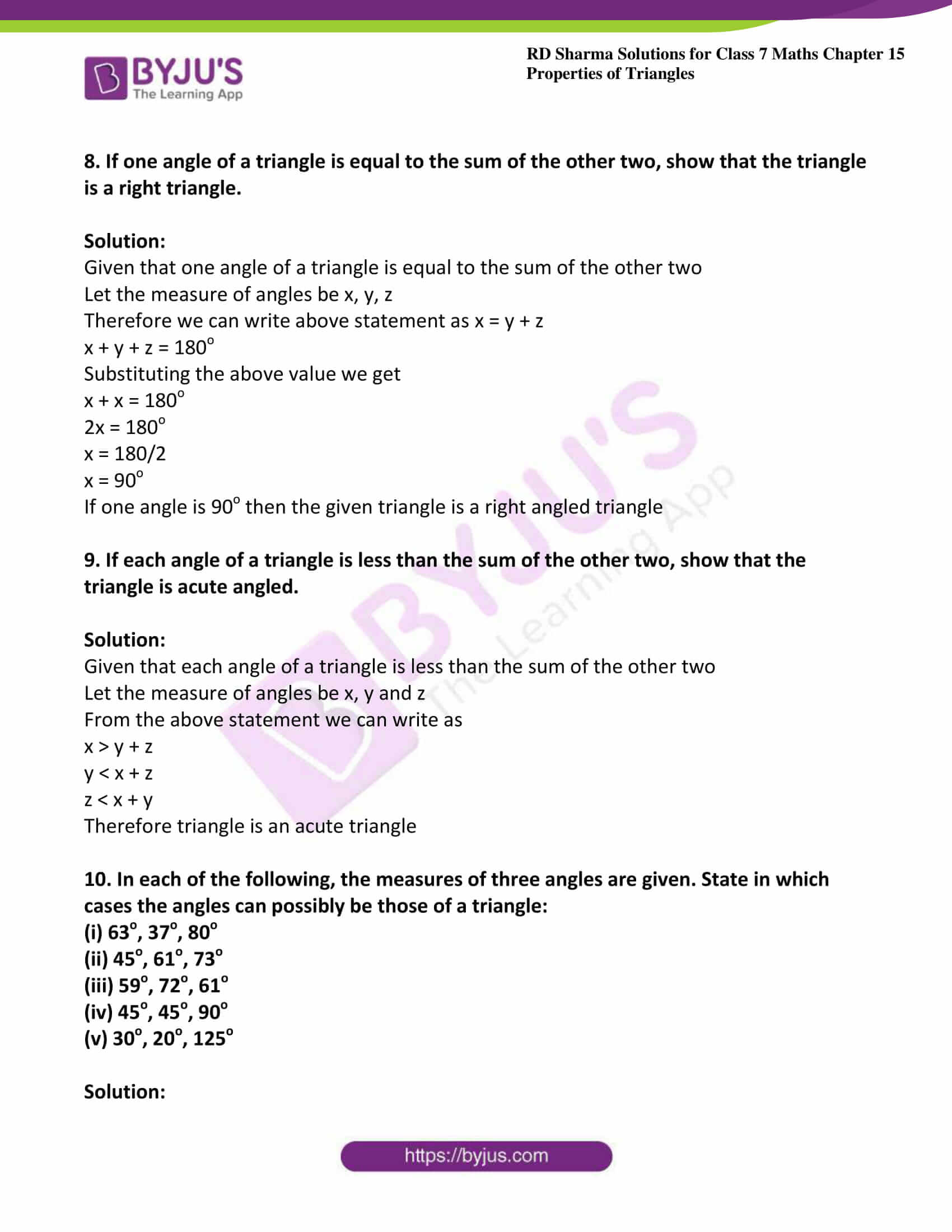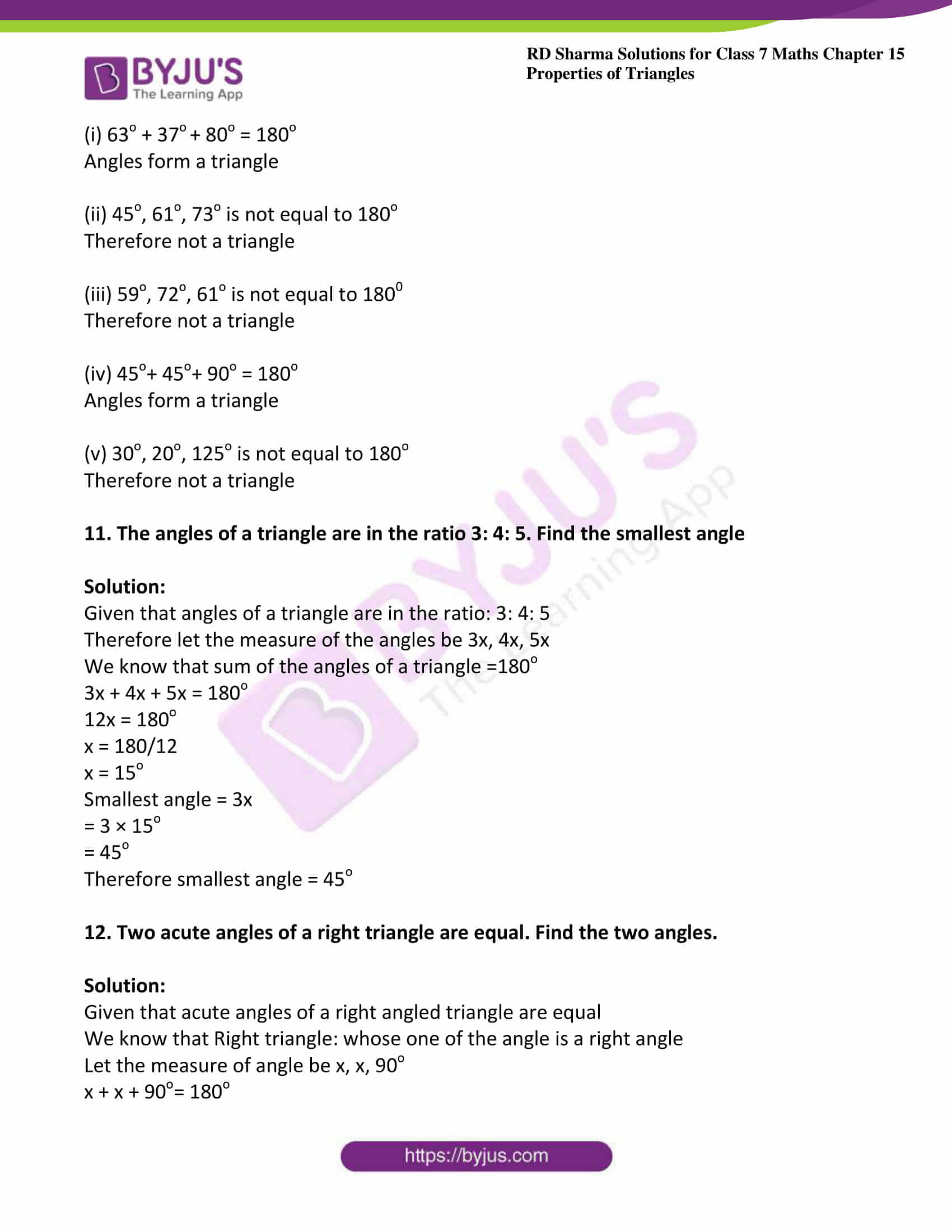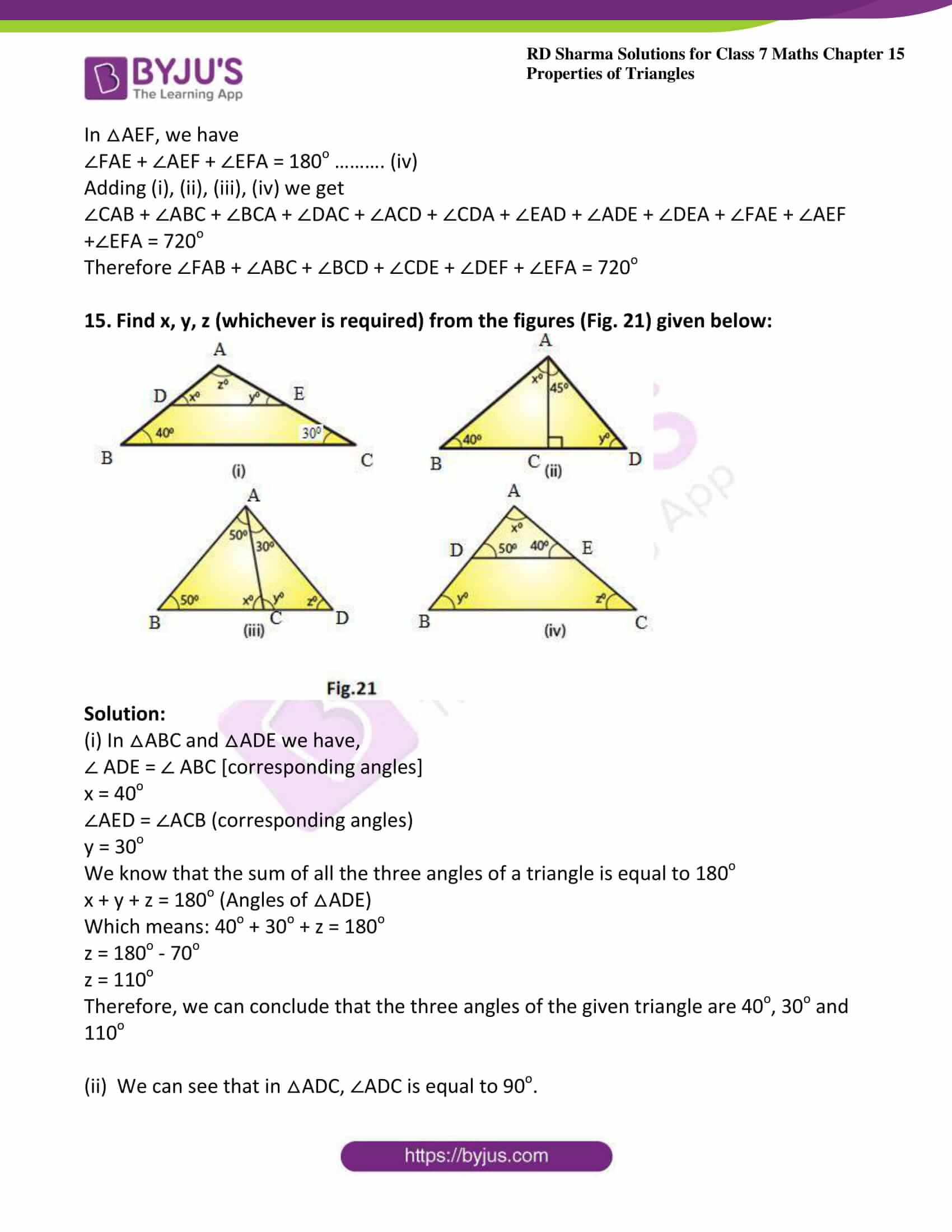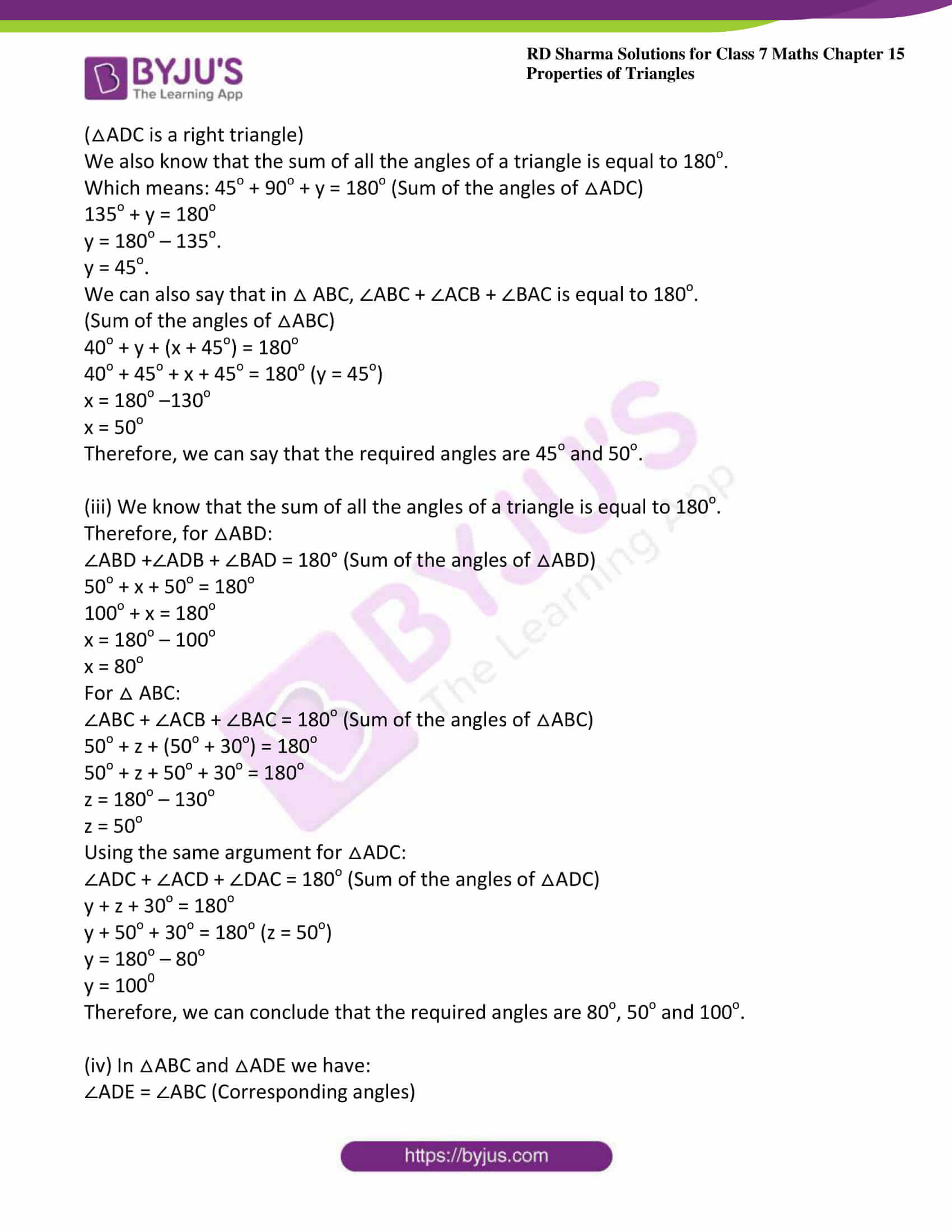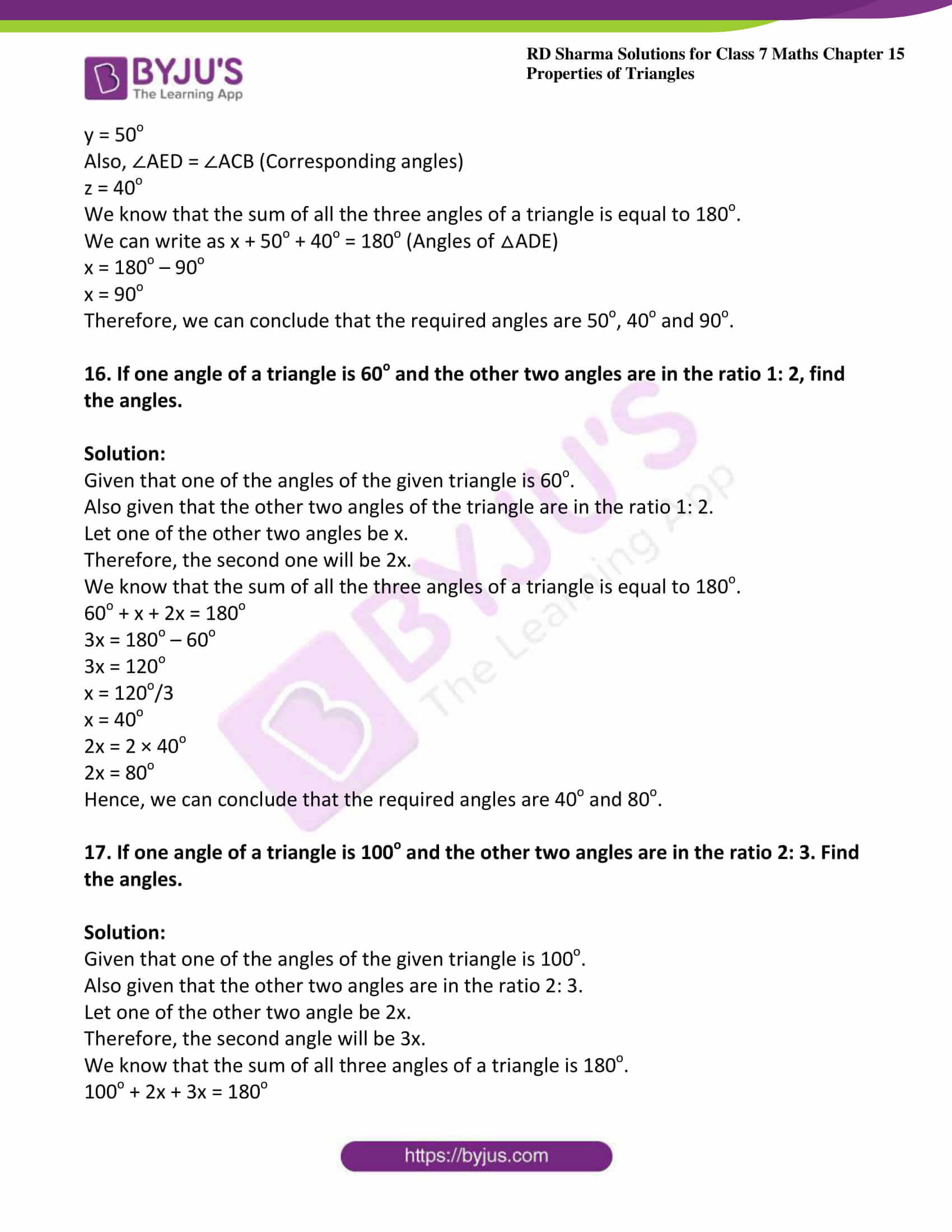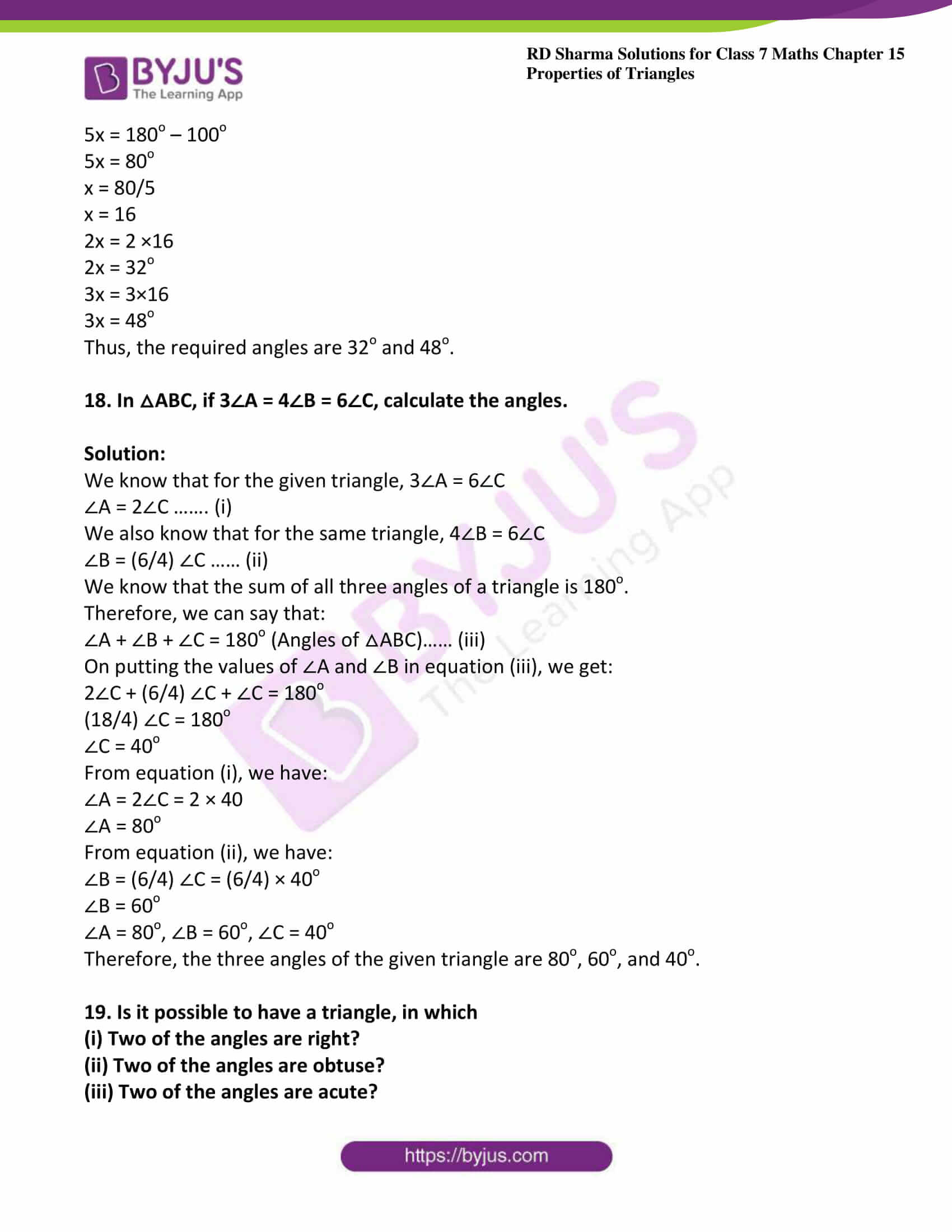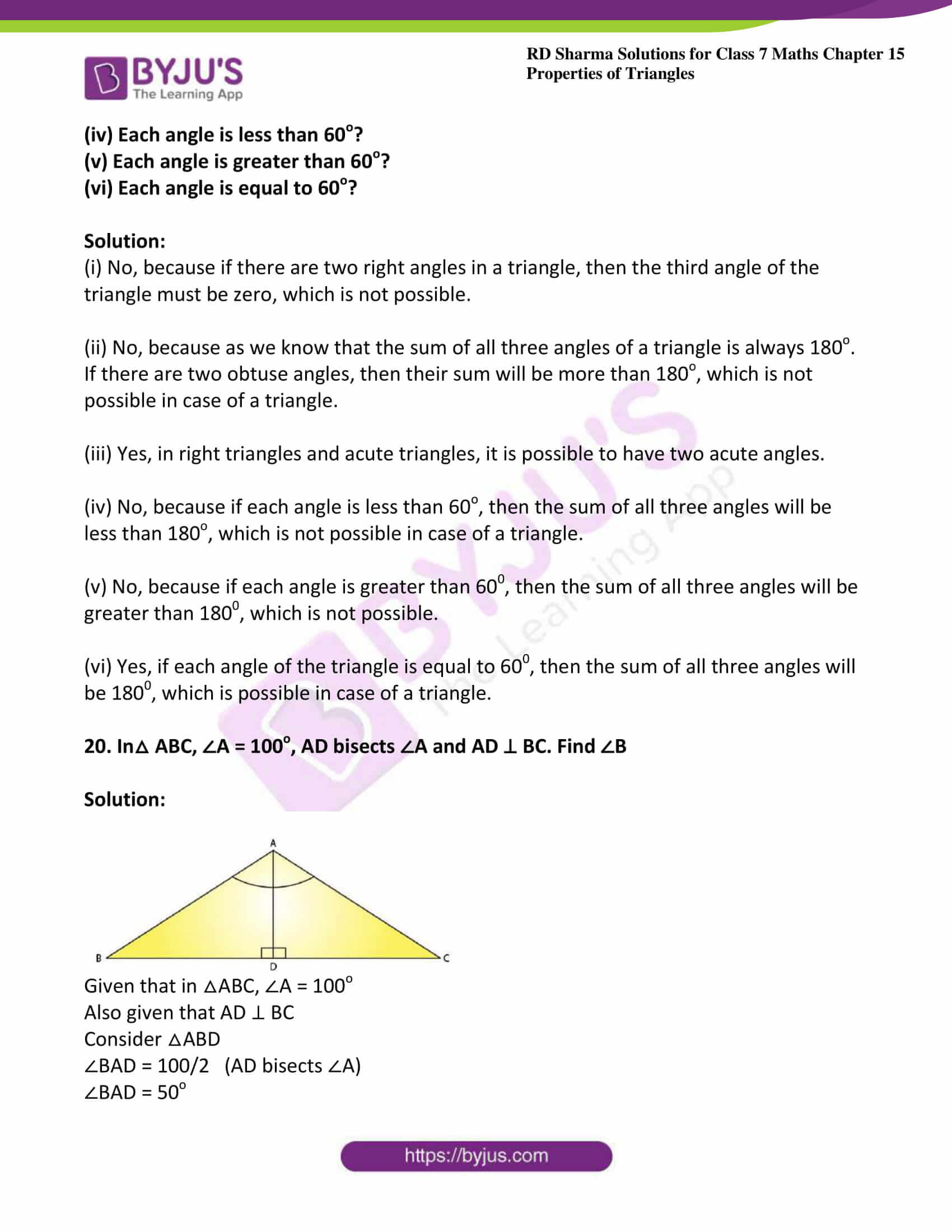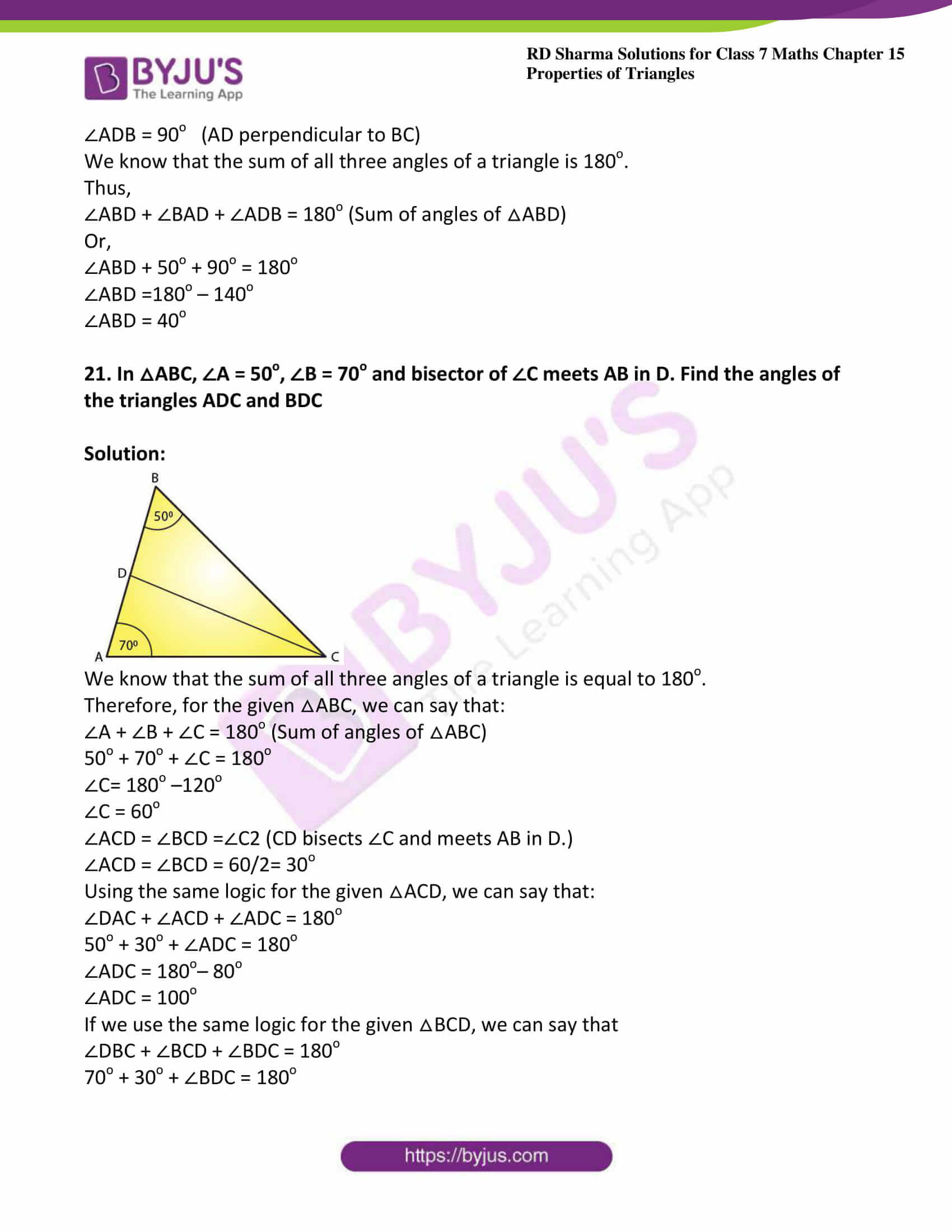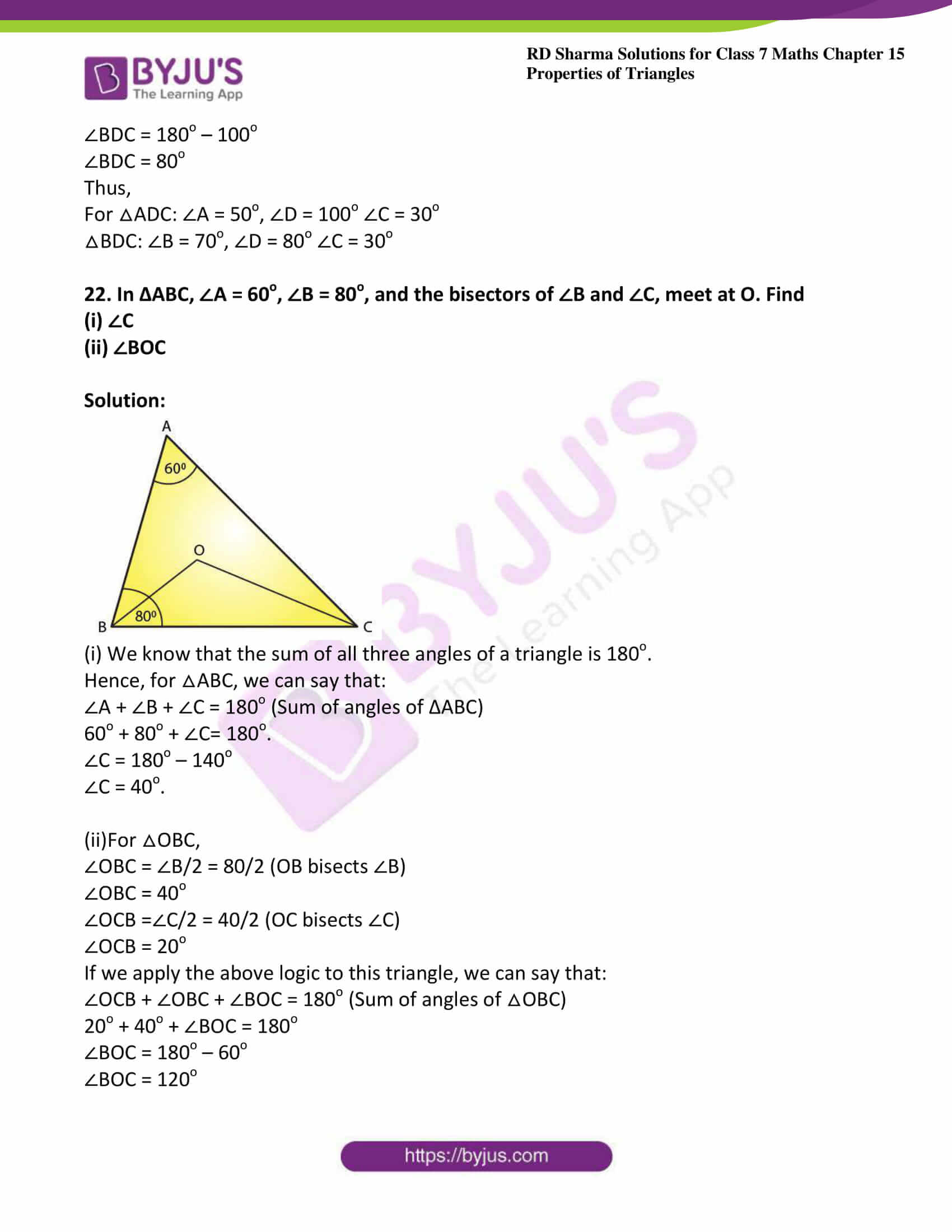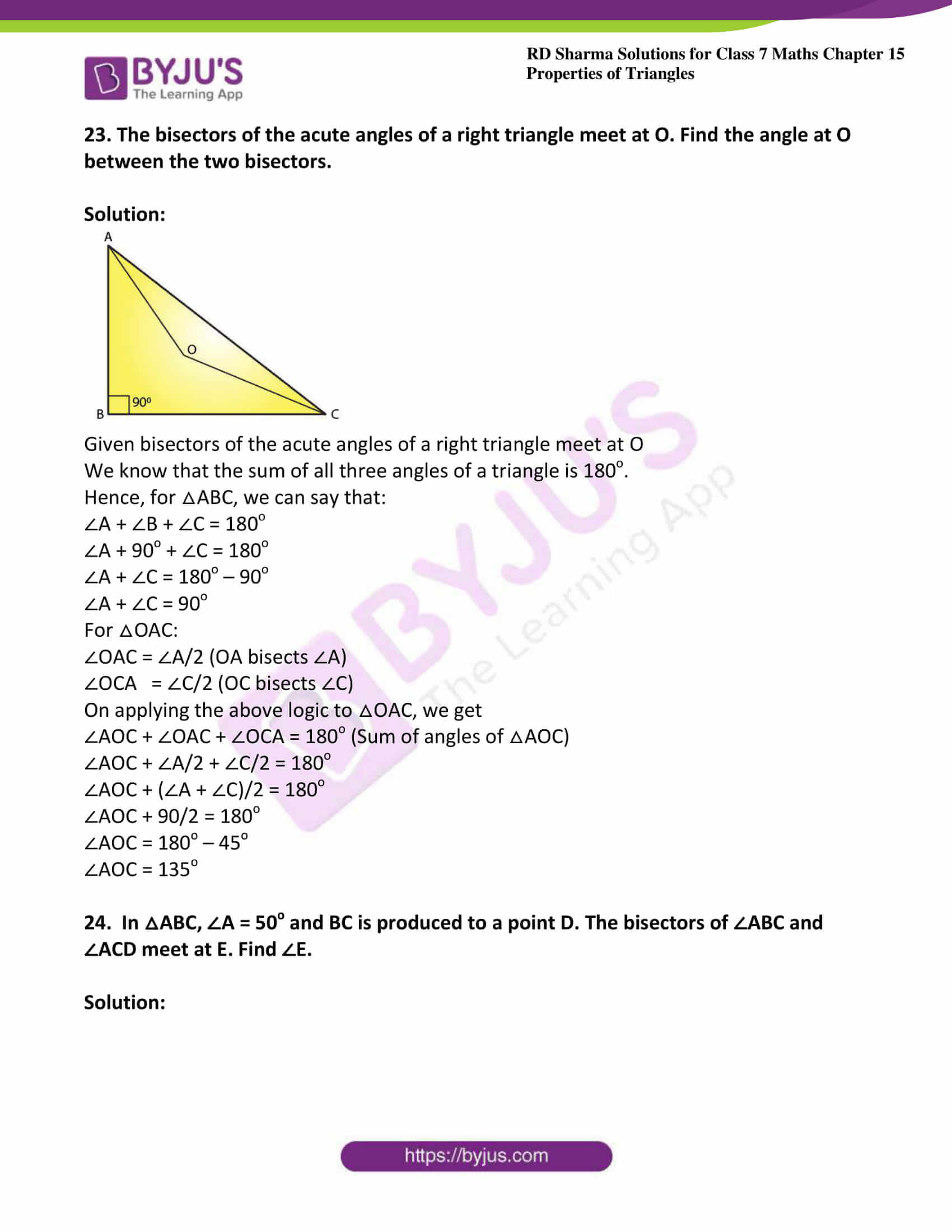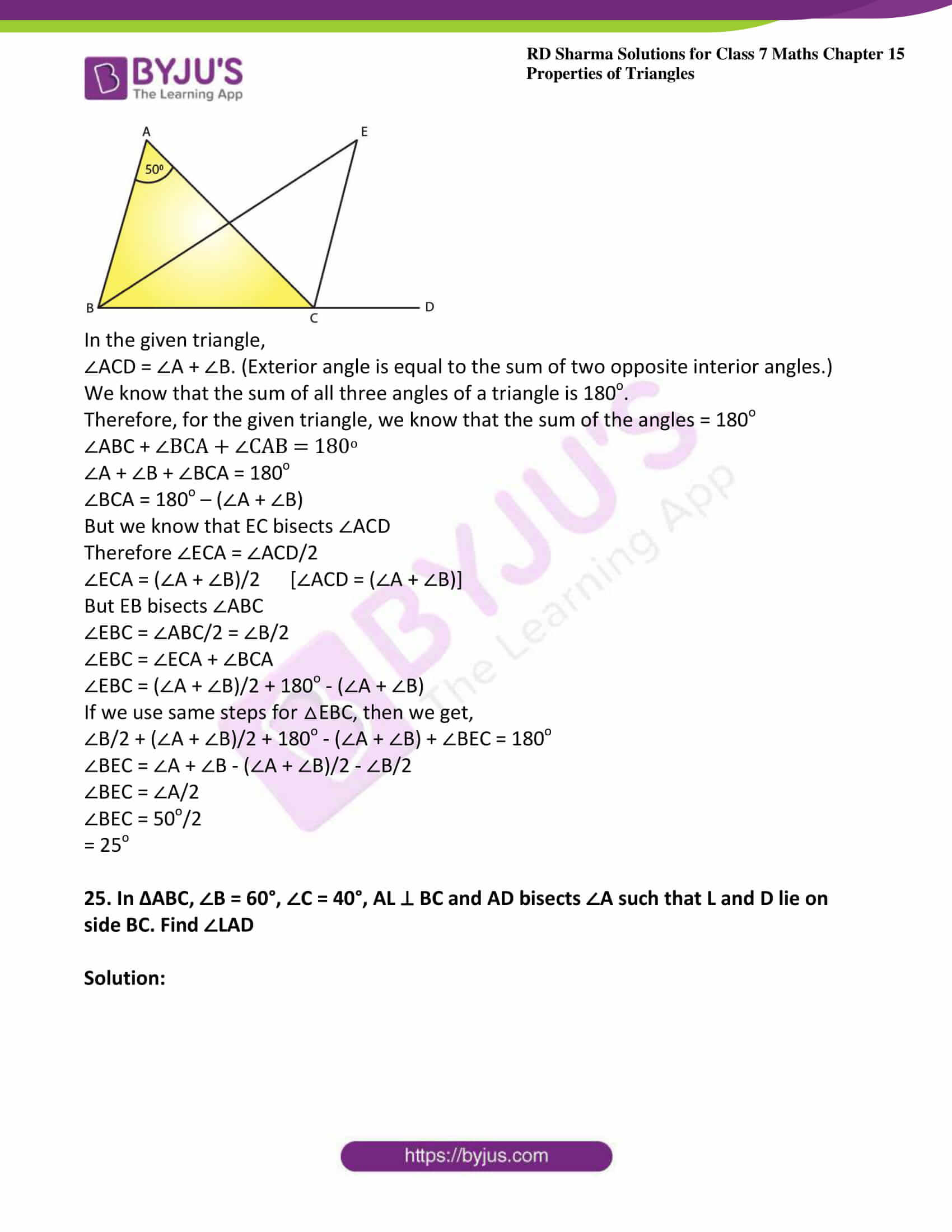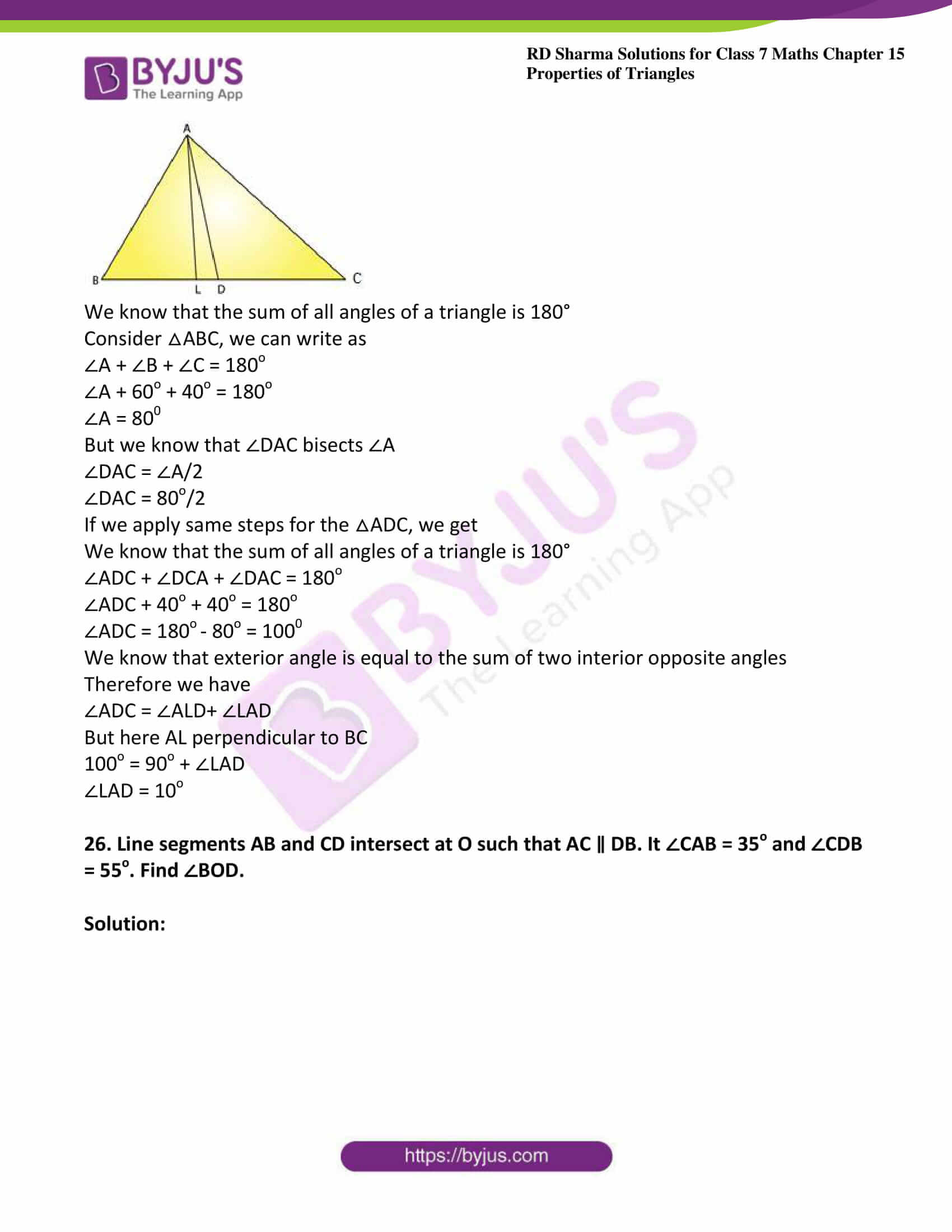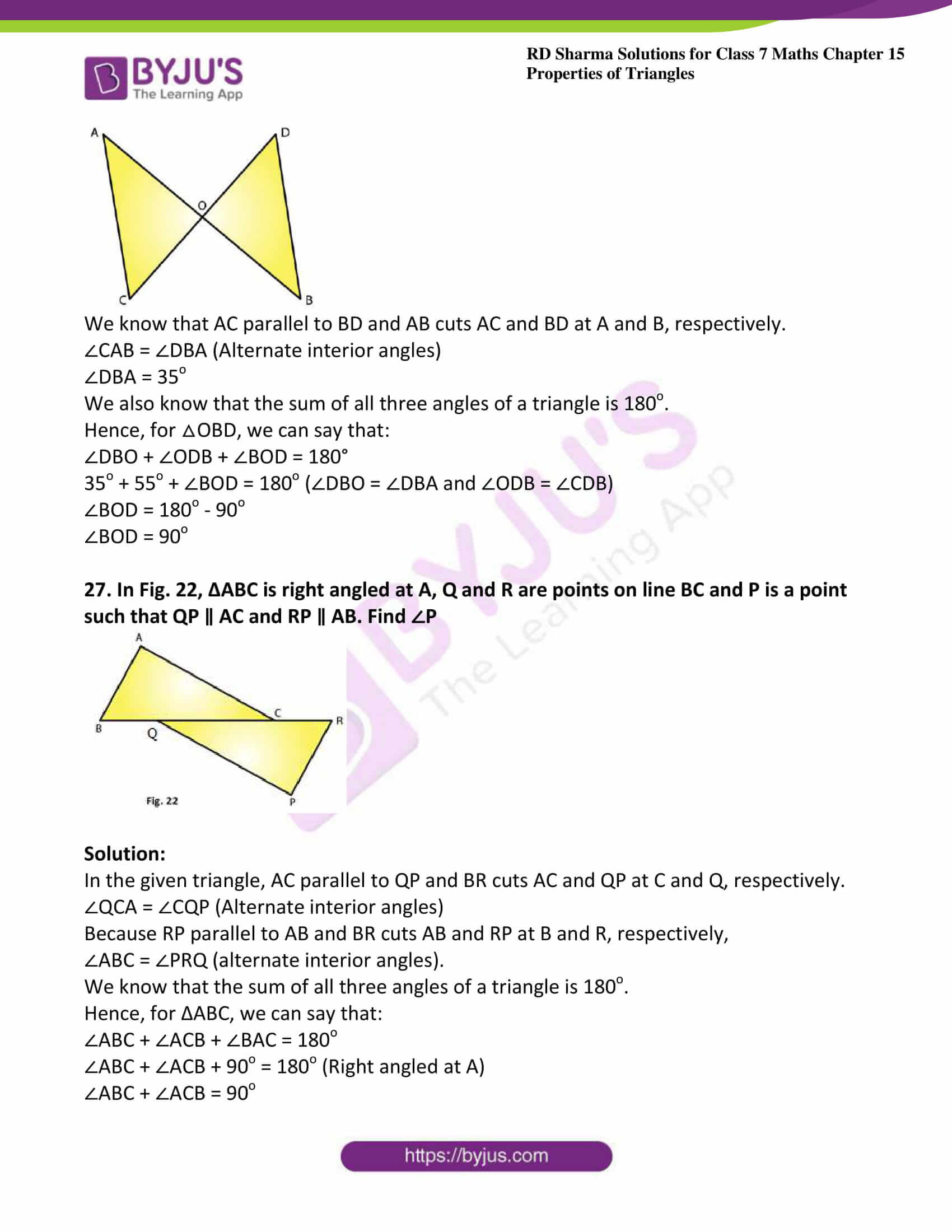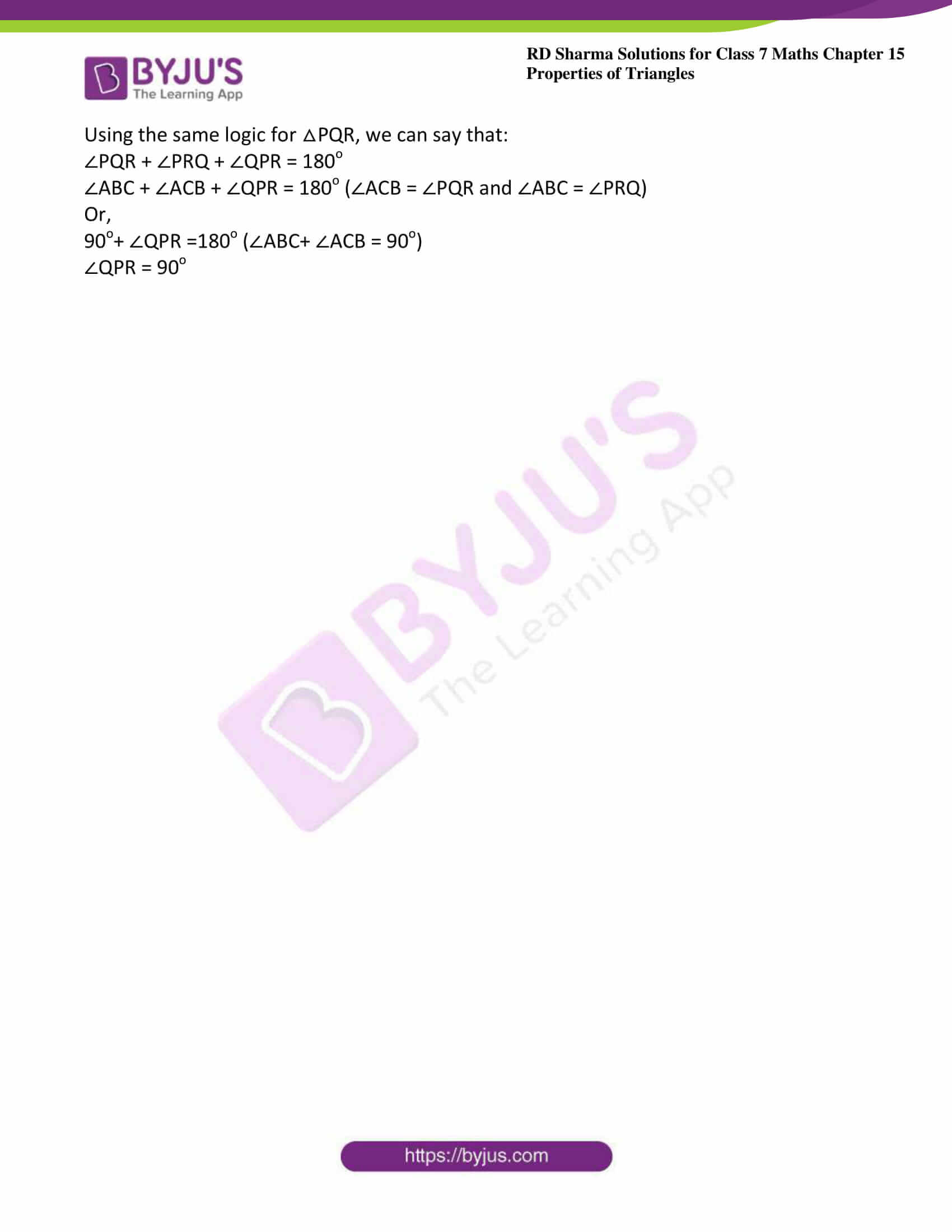### Access answers to Maths RD Sharma Solutions For Class 7 Chapter 15 – Properties of Triangles Exercise 15.2

Exercise 15.2 Page No: 15.12

1. Two angles of a triangle are of measures 150o and 30o. Find the measure of the third angle.

Solution:

Given two angles of a triangle are of measures 150o and 30o

Let the required third angle be x

We know that sum of all the angles of a triangle = 180o

150o + 30o + x = 180o

135o + x = 180o

x = 180o – 135o

x = 45o

Therefore the third angle is 45o

2. One of the angles of a triangle is 130o, and the other two angles are equal. What is the measure of each of these equal angles?

Solution:

Given one of the angles of a triangle is 130o

Also given that remaining two angles are equal

So let the second and third angle be x

We know that sum of all the angles of a triangle = 180o

130o + x + x = 180o

130o + 2x = 180o

2x = 180o – 130o

2x = 50o

x = 50/2

x = 25o

Therefore the two other angles are 25o each

3. The three angles of a triangle are equal to one another. What is the measure of each of the angles?

Solution:

Given that three angles of a triangle are equal to one another

So let the each angle be x

We know that sum of all the angles of a triangle = 180o

x + x + x = 180o

3x = 180o

x = 180/3

x = 60o

Therefore angle is 60o each

4. If the angles of a triangle are in the ratio 1: 2: 3, determine three angles.

Solution:

Given angles of the triangle are in the ratio 1: 2: 3

So take first angle as x, second angle as 2x and third angle as 3x

We know that sum of all the angles of a triangle = 180o

x + 2x + 3x = 180o

6x = 180o

x = 180/6

x = 30o

2x = 30o × 2 = 60o

3x = 30o × 3 = 90o

Therefore the first angle is 30o, second angle is 60o and third angle is 90o.

5. The angles of a triangle are (x − 40)o, (x − 20)o and (1/2 − 10)o. Find the value of x.

Solution:

Given the angles of a triangle are (x − 40)o, (x − 20)o and (1/2 − 10)o.

We know that sum of all the angles of a triangle = 180o

(x − 40)o + (x − 20)o + (1/2 − 10)o = 180o

x + x + (1/2) – 40o – 20o – 10o = 180o

x + x + (1/2) – 70o = 180o

(5x/2) = 180o + 70o

(5x/2) = 250o

x = (2/5) × 250o

x = 100o

Hence the value of x is 100o

6. The angles of a triangle are arranged in ascending order of magnitude. If the difference between two consecutive angles is 10o. Find the three angles.

Solution:

Given that angles of a triangle are arranged in ascending order of magnitude

Also given that difference between two consecutive angles is 10o

Let the first angle be x

Second angle be x + 10o

Third angle be x + 10o + 10o

We know that sum of all the angles of a triangle = 180o

x + x + 10o + x + 10o +10o = 180o

3x + 30 = 180

3x = 180 – 30

3x = 150

x = 150/3

x = 50o

First angle is 50o

Second angle x + 10o = 50 + 10 = 60o

Third angle x + 10o +10o = 50 + 10 + 10 = 70o

7. Two angles of a triangle are equal and the third angle is greater than each of those angles by 30o. Determine all the angles of the triangle

Solution:

Given that two angles of a triangle are equal

Let the first and second angle be x

Also given that third angle is greater than each of those angles by 30o

Therefore the third angle is greater than the first and second by 30o = x + 30o

The first and the second angles are equal

We know that sum of all the angles of a triangle = 180o

x + x + x + 30o = 180o

3x + 30 = 180

3x = 180 – 30

3x = 150

x = 150/3

x = 50o

Third angle = x + 30o = 50o + 30o = 80o

The first and the second angle is 50o and the third angle is 80o.

8. If one angle of a triangle is equal to the sum of the other two, show that the triangle is a right triangle.

Solution:

Given that one angle of a triangle is equal to the sum of the other two

Let the measure of angles be x, y, z

Therefore we can write above statement as x = y + z

x + y + z = 180o

Substituting the above value we get

x + x = 180o

2x = 180o

x = 180/2

x = 90o

If one angle is 90o then the given triangle is a right angled triangle

9. If each angle of a triangle is less than the sum of the other two, show that the triangle is acute angled.

Solution:

Given that each angle of a triangle is less than the sum of the other two

Let the measure of angles be x, y and z

From the above statement we can write as

x > y + z

y < x + z

z < x + y

Therefore triangle is an acute triangle

10. In each of the following, the measures of three angles are given. State in which cases the angles can possibly be those of a triangle:

(i) 63o, 37o, 80o

(ii) 45o, 61o, 73o

(iii) 59o, 72o, 61o

(iv) 45o, 45o, 90o

(v) 30o, 20o, 125o

Solution:

(i) 63o + 37o + 80o = 180o

Angles form a triangle

(ii) 45o, 61o, 73o is not equal to 180o

Therefore not a triangle

(iii) 59o, 72o, 61o is not equal to 1800

Therefore not a triangle

(iv) 45o+ 45o+ 90o = 180o

Angles form a triangle

(v) 30o, 20o, 125o is not equal to 180o

Therefore not a triangle

11. The angles of a triangle are in the ratio 3: 4: 5. Find the smallest angle

Solution:

Given that angles of a triangle are in the ratio: 3: 4: 5

Therefore let the measure of the angles be 3x, 4x, 5x

We know that sum of the angles of a triangle =180o

3x + 4x + 5x = 180o

12x = 180o

x = 180/12

x = 15o

Smallest angle = 3x

= 3 × 15o

= 45o

Therefore smallest angle = 45o

12. Two acute angles of a right triangle are equal. Find the two angles.

Solution:

Given that acute angles of a right angled triangle are equal

We know that Right triangle: whose one of the angle is a right angle

Let the measure of angle be x, x, 90o

x + x + 90o= 180o

2x = 90o

x = 90/2

x = 45o

The two angles are 45o and 45o

13. One angle of a triangle is greater than the sum of the other two. What can you say about the measure of this angle? What type of a triangle is this?

Solution:

Given one angle of a triangle is greater than the sum of the other two

Let the measure of the angles be x, y, z

From the question we can write as

x > y + z or

y > x + z   or

z > x + y

x or y or z > 90o which is obtuse

Therefore triangle is an obtuse angle

14. In the six cornered figure, (fig. 20), AC, AD and AE are joined. Find ∠FAB + ∠ABC + ∠BCD + ∠CDE + ∠DEF + ∠EFA.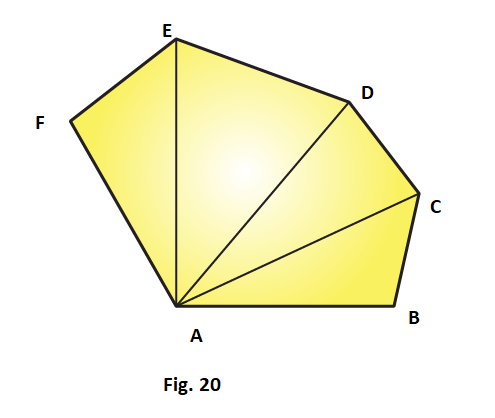Solution:

We know that sum of the angles of a triangle is 180o

Therefore in ∆ABC, we have

∠CAB + ∠ABC + ∠BCA = 180o …….. (i)

In△ ACD, we have

∠DAC + ∠ACD + ∠CDA = 180o …….. (ii)

In △AEF, we have

∠FAE + ∠AEF + ∠EFA = 180o ………. (iv)

Adding (i), (ii), (iii), (iv) we get

∠CAB + ∠ABC + ∠BCA + ∠DAC + ∠ACD + ∠CDA + ∠EAD + ∠ADE + ∠DEA + ∠FAE + ∠AEF +∠EFA = 720o

Therefore ∠FAB + ∠ABC + ∠BCD + ∠CDE + ∠DEF + ∠EFA = 720o

15. Find x, y, z (whichever is required) from the figures (Fig. 21) given below: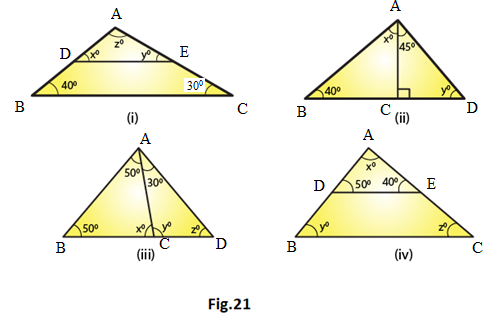Solution:

(i) In △ABC and △ADE we have,

∠ ADE = ∠ ABC [corresponding angles]

x = 40o

∠AED = ∠ACB (corresponding angles)

y = 30o

We know that the sum of all the three angles of a triangle is equal to 180o

x + y + z = 180o (Angles of △ADE)

Which means: 40o + 30o + z = 180o

z = 180o – 70o

z = 110o

Therefore, we can conclude that the three angles of the given triangle are 40o, 30o and 110o

(ii)  We can see that in △ADC, ∠ADC is equal to 90o.

We also know that the sum of all the angles of a triangle is equal to 180o.

Which means: 45o + 90o + y = 180o (Sum of the angles of △ADC)

135o + y = 180o

y = 180o – 135o.

y = 45o.

We can also say that in △ ABC, ∠ABC + ∠ACB + ∠BAC is equal to 180o.

(Sum of the angles of △ABC)

40o + y + (x + 45o) = 180o

40o + 45o + x + 45o = 180o (y = 45o)

x = 180o –130o

x = 50o

Therefore, we can say that the required angles are 45o and 50o.

(iii) We know that the sum of all the angles of a triangle is equal to 180o.

Therefore, for △ABD:

∠ABD +∠ADB + ∠BAD = 180° (Sum of the angles of △ABD)

50o + x + 50o = 180o

100o + x = 180o

x = 180o – 100o

x = 80o

For △ ABC:

∠ABC + ∠ACB + ∠BAC = 180o (Sum of the angles of △ABC)

50o + z + (50o + 30o) = 180o

50o + z + 50o + 30o = 180o

z = 180o – 130o

z = 50o

Using the same argument for △ADC:

∠ADC + ∠ACD + ∠DAC = 180o (Sum of the angles of △ADC)

y + z + 30o = 180o

y + 50o + 30o = 180o (z = 50o)

y = 180o – 80o

y = 1000

Therefore, we can conclude that the required angles are 80o, 50o and 100o.

(iv) In △ABC and △ADE we have:

y = 50o

Also, ∠AED = ∠ACB (Corresponding angles)

z = 40o

We know that the sum of all the three angles of a triangle is equal to 180o.

We can write as x + 50o + 40o = 180o (Angles of △ADE)

x = 180o – 90o

x = 90o

Therefore, we can conclude that the required angles are 50o, 40o and 90o.

16. If one angle of a triangle is 60o and the other two angles are in the ratio 1: 2, find the angles.

Solution:

Given that one of the angles of the given triangle is 60o.

Also given that the other two angles of the triangle are in the ratio 1: 2.

Let one of the other two angles be x.

Therefore, the second one will be 2x.

We know that the sum of all the three angles of a triangle is equal to 180o.

60o + x + 2x = 180o

3x = 180o – 60o

3x = 120o

x = 120o/3

x = 40o

2x = 2 × 40o

2x = 80o

Hence, we can conclude that the required angles are 40o and 80o.

17. If one angle of a triangle is 100o and the other two angles are in the ratio 2: 3. Find the angles.

Solution:

Given that one of the angles of the given triangle is 100o.

Also given that the other two angles are in the ratio 2: 3.

Let one of the other two angle be 2x.

Therefore, the second angle will be 3x.

We know that the sum of all three angles of a triangle is 180o.

100o + 2x + 3x = 180o

5x = 180o – 100o

5x = 80o

x = 80/5

x = 16

2x = 2 ×16

2x = 32o

3x = 3×16

3x = 48o

Thus, the required angles are 32o and 48o.

18. In △ABC, if 3∠A = 4∠B = 6∠C, calculate the angles.

Solution:

We know that for the given triangle, 3∠A = 6∠C

∠A = 2∠C ……. (i)

We also know that for the same triangle, 4∠B = 6∠C

∠B = (6/4) ∠C …… (ii)

We know that the sum of all three angles of a triangle is 180o.

Therefore, we can say that:

∠A + ∠B + ∠C = 180o (Angles of △ABC)…… (iii)

On putting the values of ∠A and ∠B in equation (iii), we get:

2∠C + (6/4) ∠C + ∠C = 180o

(18/4) ∠C = 180o

∠C = 40o

From equation (i), we have:

∠A = 2∠C = 2 × 40

∠A = 80o

From equation (ii), we have:

∠B = (6/4) ∠C = (6/4) × 40o

∠B = 60o

∠A = 80o, ∠B = 60o, ∠C = 40o

Therefore, the three angles of the given triangle are 80o, 60o, and 40o.

19. Is it possible to have a triangle, in which

(i) Two of the angles are right?

(ii) Two of the angles are obtuse?

(iii) Two of the angles are acute?

(iv) Each angle is less than 60o?

(v) Each angle is greater than 60o?

(vi) Each angle is equal to 60o?

Solution:

(i) No, because if there are two right angles in a triangle, then the third angle of the triangle must be zero, which is not possible.

(ii) No, because as we know that the sum of all three angles of a triangle is always 180o. If there are two obtuse angles, then their sum will be more than 180o, which is not possible in case of a triangle.

(iii) Yes, in right triangles and acute triangles, it is possible to have two acute angles.

(iv) No, because if each angle is less than 60o, then the sum of all three angles will be less than 180o, which is not possible in case of a triangle.

(v) No, because if each angle is greater than 600, then the sum of all three angles will be greater than 1800, which is not possible.

(vi) Yes, if each angle of the triangle is equal to 600, then the sum of all three angles will be 1800, which is possible in case of a triangle.

20. In△ ABC, ∠A = 100o, AD bisects ∠A and AD ⊥ BC. Find ∠B

Solution: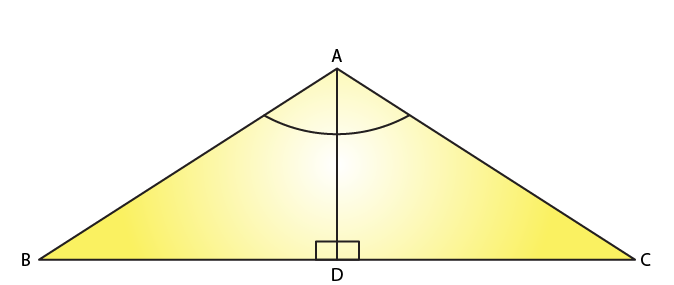Given that in △ABC, ∠A = 100o

Also given that AD ⊥ BC

Consider △ABD

We know that the sum of all three angles of a triangle is 180o.

Thus,

∠ABD + ∠BAD + ∠ADB = 180o (Sum of angles of △ABD)

Or,

∠ABD + 50o + 90o = 180o

∠ABD =180o – 140o

∠ABD = 40o

21. In △ABC, ∠A = 50o, ∠B = 70o and bisector of ∠C meets AB in D. Find the angles of the triangles ADC and BDC

Solution: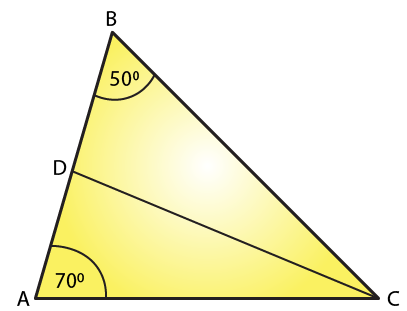We know that the sum of all three angles of a triangle is equal to 180o.

Therefore, for the given △ABC, we can say that:

∠A + ∠B + ∠C = 180o (Sum of angles of △ABC)

50o + 70o + ∠C = 180o

∠C= 180o –120o

∠C = 60o

∠ACD = ∠BCD =∠C2 (CD bisects ∠C and meets AB in D.)

∠ACD = ∠BCD = 60/2= 30o

Using the same logic for the given △ACD, we can say that:

∠DAC + ∠ACD + ∠ADC = 180o

50o + 30o + ∠ADC = 180o

If we use the same logic for the given △BCD, we can say that

∠DBC + ∠BCD + ∠BDC = 180o

70o + 30o + ∠BDC = 180o

∠BDC = 180o – 100o

∠BDC = 80o

Thus,

For △ADC: ∠A = 50o, ∠D = 100o ∠C = 30o

△BDC: ∠B = 70o, ∠D = 80o ∠C = 30o

22. In ∆ABC, ∠A = 60o, ∠B = 80o, and the bisectors of ∠B and ∠C, meet at O. Find

(i) ∠C

(ii) ∠BOC

Solution: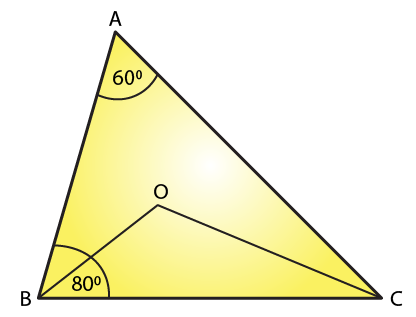(i) We know that the sum of all three angles of a triangle is 180o.

Hence, for △ABC, we can say that:

∠A + ∠B + ∠C = 180o (Sum of angles of ∆ABC)

60o + 80o + ∠C= 180o.

∠C = 180o – 140o

∠C = 40o.

(ii)For △OBC,

∠OBC = ∠B/2 = 80/2 (OB bisects ∠B)

∠OBC = 40o

∠OCB =∠C/2 = 40/2 (OC bisects ∠C)

∠OCB = 20o

If we apply the above logic to this triangle, we can say that:

∠OCB + ∠OBC + ∠BOC = 180o (Sum of angles of △OBC)

20o + 40o + ∠BOC = 180o

∠BOC = 180o – 60o

∠BOC = 120o

23. The bisectors of the acute angles of a right triangle meet at O. Find the angle at O between the two bisectors.

Solution: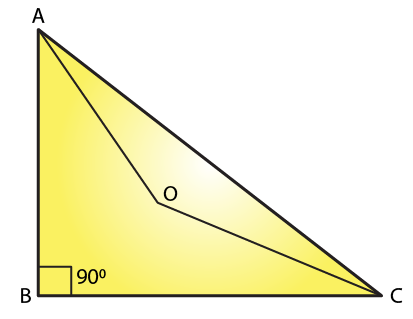Given bisectors of the acute angles of a right triangle meet at O

We know that the sum of all three angles of a triangle is 180o.

Hence, for △ABC, we can say that:

∠A + ∠B + ∠C = 180o

∠A + 90o + ∠C = 180o

∠A + ∠C = 180o – 90o

∠A + ∠C = 90o

For △OAC:

∠OAC = ∠A/2 (OA bisects ∠A)

∠OCA   = ∠C/2 (OC bisects ∠C)

On applying the above logic to △OAC, we get

∠AOC + ∠OAC + ∠OCA = 180o (Sum of angles of △AOC)

∠AOC + ∠A/2 + ∠C/2 = 180o

∠AOC + (∠A + ∠C)/2 = 180o

∠AOC + 90/2 = 180o

∠AOC = 180o – 45o

∠AOC = 135o

24.  In △ABC, ∠A = 50o and BC is produced to a point D. The bisectors of ∠ABC and ∠ACD meet at E. Find ∠E.

Solution: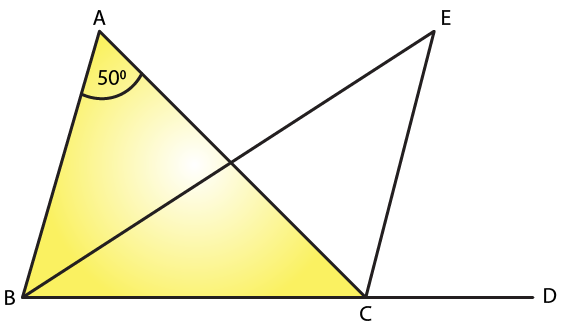In the given triangle,

∠ACD = ∠A + ∠B. (Exterior angle is equal to the sum of two opposite interior angles.)

We know that the sum of all three angles of a triangle is 180o.

Therefore, for the given triangle, we know that the sum of the angles = 180o

∠ABC + ∠BCA + ∠CAB = 180o

∠A + ∠B + ∠BCA = 180o

∠BCA = 180o – (∠A + ∠B)

But we know that EC bisects ∠ACD

Therefore ∠ECA = ∠ACD/2

∠ECA = (∠A + ∠B)/2 [∠ACD = (∠A + ∠B)]

But EB bisects ∠ABC

∠EBC = ∠ABC/2 = ∠B/2

∠EBC = ∠ECA + ∠BCA

∠EBC = (∠A + ∠B)/2 + 180o – (∠A + ∠B)

If we use same steps for △EBC, then we get,

∠B/2 + (∠A + ∠B)/2 + 180o – (∠A + ∠B) + ∠BEC = 180o

∠BEC = ∠A + ∠B – (∠A + ∠B)/2 – ∠B/2

∠BEC = ∠A/2

∠BEC = 50o/2

= 25o

25. In ∆ABC, ∠B = 60°, ∠C = 40°, AL ⊥ BC and AD bisects ∠A such that L and D lie on side BC. Find ∠LAD

Solution: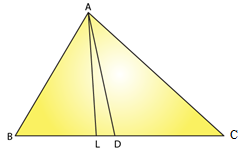We know that the sum of all angles of a triangle is 180°

Consider △ABC, we can write as

∠A + ∠B + ∠C = 180o

∠A + 60o + 40o = 180o

∠A = 800

But we know that ∠DAC bisects ∠A

∠DAC = ∠A/2

∠DAC = 80o/2

If we apply same steps for the △ADC, we get

We know that the sum of all angles of a triangle is 180°

∠ADC + ∠DCA + ∠DAC = 180o

∠ADC + 40o + 40o = 180o

∠ADC = 180o – 80o = 1000

We know that exterior angle is equal to the sum of two interior opposite angles

Therefore we have

But here AL perpendicular to BC

26. Line segments AB and CD intersect at O such that AC ∥ DB. It ∠CAB = 35o and ∠CDB = 55o. Find ∠BOD.

Solution: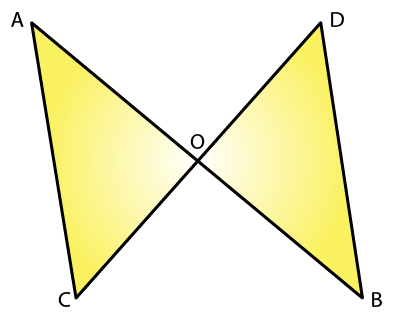We know that AC parallel to BD and AB cuts AC and BD at A and B, respectively.

∠CAB = ∠DBA (Alternate interior angles)

∠DBA = 35o

We also know that the sum of all three angles of a triangle is 180o.

Hence, for △OBD, we can say that:

∠DBO + ∠ODB + ∠BOD = 180°

35o + 55o + ∠BOD = 180o (∠DBO = ∠DBA and ∠ODB = ∠CDB)

∠BOD = 180o – 90o

∠BOD = 90o

27. In Fig. 22, ∆ABC is right angled at A, Q and R are points on line BC and P is a point such that QP ∥ AC and RP ∥ AB. Find ∠P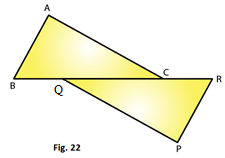Solution:

In the given triangle, AC parallel to QP and BR cuts AC and QP at C and Q, respectively.

∠QCA = ∠CQP (Alternate interior angles)

Because RP parallel to AB and BR cuts AB and RP at B and R, respectively,

∠ABC = ∠PRQ (alternate interior angles).

We know that the sum of all three angles of a triangle is 180o.

Hence, for ∆ABC, we can say that:

∠ABC + ∠ACB + ∠BAC = 180o

∠ABC + ∠ACB + 90o = 180o (Right angled at A)

∠ABC + ∠ACB = 90o

Using the same logic for △PQR, we can say that:

∠PQR + ∠PRQ + ∠QPR = 180o

∠ABC + ∠ACB + ∠QPR = 180o (∠ACB = ∠PQR and ∠ABC = ∠PRQ)

Or,

90o+ ∠QPR =180o (∠ABC+ ∠ACB = 90o)

∠QPR = 90o